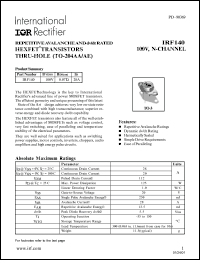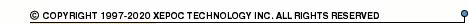More than12 565 035 queries processedIRF140 series datasheets. Manufacturer: International Rectifier.

 IRF140 Repetitive avalanche and dv/dt rated HEXFET transistor thru-hole. BVDSS = 100V, RDS(on) = 0.077 Ohm, ID = 28A. in 3-pin TO-3 package. Operational temperature range from -55°C to 175°C. Datasheet*) IRF140 Repetitive avalanche and dv/dt rated HEXFET transistor thru-hole. BVDSS = 100V, RDS(on) = 0.077 Ohm, ID = 28A. in 3-pin TO-204AA package. Operational temperature range from -55°C to 175°C. Datasheet*) IRF140 Repetitive avalanche and dv/dt rated HEXFET transistor thru-hole. BVDSS = 100V, RDS(on) = 0.077 Ohm, ID = 28A. in 3-pin TO-204AE package. Operational temperature range from -55°C to 175°C. Datasheet*) IRF1404 HEXFET power MOSFET. VDSS = 40V, RDS(on) = 0.004 Ohm, ID = 162A. in 3-pin TO-220AB package. Operational temperature range from -55°C to 175°C. Datasheet*) IRF1404L HEXFET power MOSFET. VDSS = 40V, RDS(on) = 0.004 Ohm, ID = 162A. in 3-pin TO-262 package. Operational temperature range from -55°C to 175°C. Datasheet*) IRF1404S HEXFET power MOSFET. VDSS = 40V, RDS(on) = 0.004 Ohm, ID = 162A. in 3-pin DDPak package. Operational temperature range from -55°C to 175°C. Datasheet*) IRF1405 HEXFET power MOSFET. VDSS = 55V, RDS(on) = 5.3 mOhm, ID = 169A. in 3-pin TO-220AB package. Operational temperature range from -55°C to 175°C. Datasheet*) IRF1405L HEXFET power MOSFET. VDSS = 55V, RDS(on) = 5.3 mOhm, ID = 131A. in 3-pin TO-262 package. Operational temperature range from -55°C to 175°C. Datasheet*) IRF1405S HEXFET power MOSFET. VDSS = 55V, RDS(on) = 5.3 mOhm, ID = 131A. in 3-pin DDPak package. Operational temperature range from -55°C to 175°C. Datasheet*) IRF1407 HEXFET power MOSFET. VDSS = 75V, RDS(on) = 0.0078 Ohm, ID = 130A. in 3-pin TO-220AB package. Operational temperature range from -55°C to 175°C. Datasheet*) IRF1407L HEXFET power MOSFET. VDSS = 75V, RDS(on) = 0.0078 Ohm, ID = 100A. in 3-pin TO-262 package. Operational temperature range from -55°C to 175°C. Datasheet*) IRF1407S HEXFET power MOSFET. VDSS = 75V, RDS(on) = 0.0078 Ohm, ID = 100A. in 3-pin DDPak package. Operational temperature range from -55°C to 175°C. Datasheet*) There are other manufacturers for this components. For more information please check IRF140 (Fairchild Semiconductor) IRF140 (Intersil Corp.) IRF140 (Semelab Plc.) IRF140 (Samsung Electronic)